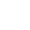# 公設化集合論的奧秘(18) 優雅的等式〡R〡=〡P(N)〡=〡2^N〡

2015/04/02 | | 標籤：

f: R → P(Q)

r → r

〡A〡=〡B〡

θ: 2N → R

F: {0, 1, 2, 3, 4…} → {0, 1}

θ: 2N → R

f →  r

θ: 2N →(0, 1)

f →  0.a0a1a2a3a4…an

f(0) = 1, f(1) = 0, f(2) = 0, f(3) = 0, f(4) = 0,  … f(n) = 0 …

〡2N 〡= 〡P(N)〡≤ 〡R〡

〡N〡= 〡Q〡= ℵ0

〡R〡= 〡P(N)〡=〡2N〡= ℵ1

## 關於作者